Example 1: Add: 52.3 + 973.41

Analysis: Let's use our knowledge of mixed numbers to help us analyze this problem.

52.3 =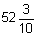and  973.41=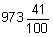Recall that a decimal number can have a whole-number part and a fractional part. When adding decimals, you must first line up all the decimal points in a column. Lining up the decimal points ensures that each digit is in the proper place-value position. Once each digit is in the proper place-value position, the whole-number parts are lined up with each other, and the fractional parts are lined up with each other. This is shown in the table below.

 PLACE VALUE AND DECIMALS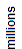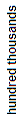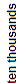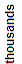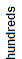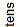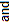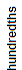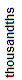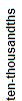5 2 . 3 9 7 3 . 4 1 ----------- Whole-Number Part ----------- ---------- Fractional Part -----------

Now that we have lined up the decimal points, you will notice that the numbers being added do not have the same number of decimal digits. Let's look at this problem on paper without a place-value chart.

52.3

+  973.41

You can write an extra zero to the right of the last digit of the first decimal so that both decimals have the same number of decimal digits.

52.30

+  973.41

Just as with whole numbers, start on the right, and add each column in turn. Note that you are adding digits in the same place-value position.

52.30

+  973.41
1025.71

Place the decimal point in the sum.

52.30

+  973.41
1025.71

Answer: The sum of 52.3 and 973.41 is 1025.71.

Example 2: Add: 0.078 + 3.09 + 0.6

0.078

3.090

+  0.600

Analysis: If you need to carry (i.e. if a columns adds up to more than 9), remember to add the tens digit of that column to the next column.

1

0.078

3.090

+  0.600
3.768

Answer: The sum of 0.078 and 3.09 and 0.6 is 3.768.Example 3: Add: \$77.23 and \$88

Analysis: Each of these numbers represents money; however, the second is written as a whole number. Change the second number so that it has two decimal digits, and then perform the addition.

\$  77.23

+ \$  88.00
\$165.23

Answer: The sum of \$77.23 and \$88 is \$165.23.Procedure: To add decimals, follow these steps:

1. Line up all the decimal points in a column.
2. When needed, write one or more extra zeros to the right so that both decimals have the same number of decimal digits.
3. Start on the right, and add each column in turn. (Add digits in the same place-value position.)
4. If you need to carry, remember to add the tens digit of that column to the next column.
5. Place the decimal point in the sum.

Example 4: Add: 28.5 + 34.5

Analysis: You will need to carry more than once. Accordingly, the addition will be divided into three steps to show the process of carrying.

 1                   1 1               1 1    28.5               28.5             28.5+ 34.5            + 34.5          + 34.5        .0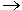3.063.0

Answer: The sum of 28.5 and 34.5 is 63.

Example 5: Add: 3.986 + 37 + 25.902

 1                     1 1                   1 1      3.986                3.986             3.986    37.000               37.000           37.000+ 25.902            + 25.902         +25.902        .8886.88866.888

Answer: The sum of 28.5 and 34.5 is 66.888.

Example 6: Add: \$12.95 + \$67.89 + \$54.55

 1                   2  1               1  2  1    \$  12.95          \$  12.95          \$  12.95    \$  67.89          \$  67.89          \$  67.89+ \$  54.55       + \$  54.55      +  \$  54.55    .39\$    5.39\$135.39

Answer: The sum of \$12.95 and \$67.89 and \$54.55 is \$135.39.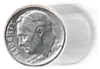Example 7: A dime rolled 5.8794 cm and then rolled 7.48 cm. How far did it roll altogether?

 1                     1  1                  1 1  1    5.8794               5.8794             5.8794+ 7.4800            + 7.4800          + 7.4800      .35943.359413.3594

Answer: The sum of 5.8794 cm and 7.48 cm is 13.3594 cm.

Summary: When adding decimals, you must first line up all the decimal points in a column. Lining up the decimal points ensures that each digit is in the proper place-value position. You can then add digits in the same place-value position to find the sum.

### Exercises

Directions: Read each question below. You may use paper and pencil to help you add. Click once in an ANSWER BOX and type in your answer; then click ENTER. After you click ENTER, a message will appear in the RESULTS BOX to indicate whether your answer is correct or incorrect. To start over, click CLEAR.